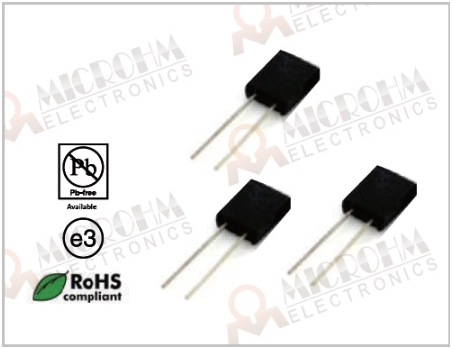Tel:400-803-9333
• Welcome to Microhm

#Position：Home » Technical Articles

# Resistance changes with temperature

Writer：Microhm Page View：Date：2020-01-19
The temperature coefficient of resistance, or TCR, is one of the main used parameters to characterize a resistor. The TCR defines the change in resistance as a function of the ambient temperature. The common way to express the TCR is in ppm/°C, which stands for parts per million per centigrade degree. Microhm Electronics can provide resistors with TCR as low as 1ppm/K.Positive or Negative Temperature Coefficient of Resistance?
Resistors are available with a TCR that is negative, positive, or stable over a certain temperature range. Choosing the right resistor could prevent the need for temperature compensation. In some applications it is desired to have a large TCR, for example to measure temperature. Resistors for these applications are called thermistors, and can have a positive (PTC) or negative temperature coefficient (NTC).

Measuring methods for the TCR
The temperature coefficient of resistance for a resistor is determined by measuring the resistances values over an appropriate temperature range. The TCR is calculated as the average slope of the resistance value over this interval. This is accurate for linear relations, since the TCR is constant at every temperature. However, many materials have a non linear coefficient. For Nichrome for example, a popular alloy for resistors, the relation between temperature and TCR is not linear. Because the TCR is calculated as average slope, it is therefore very important to specify the TCR as well as the temperature interval. The way to measure TCR is standardized in MIL-STD-202 Method 304. With this method, TCR is calculated for the range between -55°C and 25°C and between 25°C and 125°C.Values for TCR depend on metal material purity and temperature. Microhm Electronics use metal materials of relevant company to guarantee the quality of all kinds of resistors, especially for metal foil resistors and full metal resistors.
Keywords：